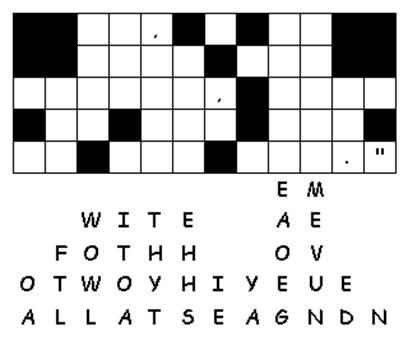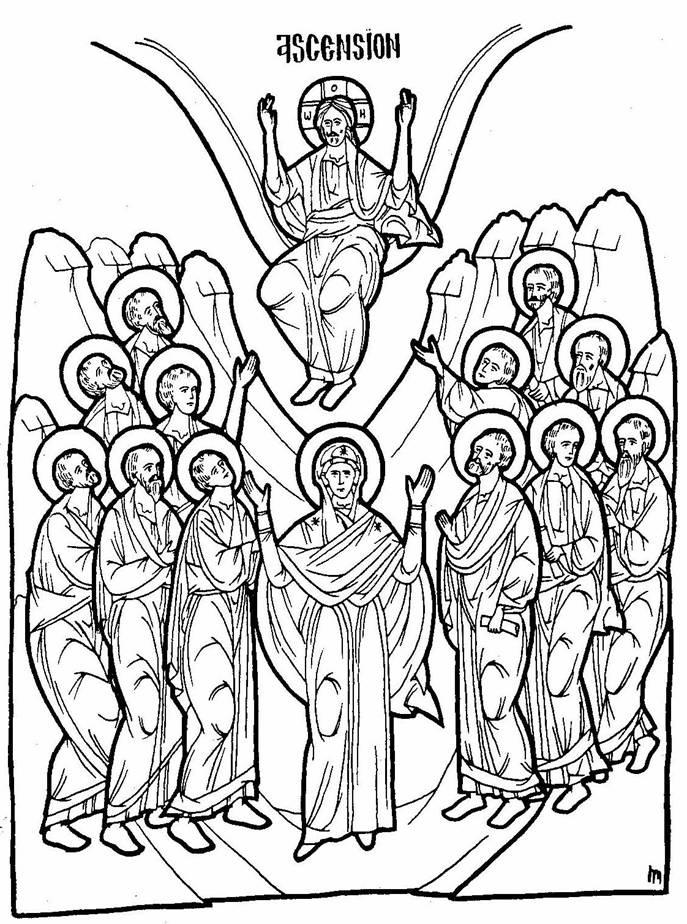# Dr. Pat's Orthodox Super Sunday School Curriculum

## Ascension

Ascension

Home Activities:

• Discuss the 3 stages of the life cycle of the butterfly:
1. Crawl on floor like larva.
2. Lie quietly on floor with eyes closed (cocoon)
3. Fly around the room like a butterfly.
• Place the icon of the feast in a prominent location.
• Word Puzzle for older children.
• Read the story at bedtime each night.

Prayer: Dear Jesus, help me to be kind and gentle like the Butterfly.

# An Ascension Promise# A Hidden Ascension Command from the Lord

`S G O T H E R M M E L A S U R E J E F N `
`O E R E A N O D M A K E D I S C I P O P `
`B L R E S U O F A L L T H E N A T I I R `
`O L N U N S B A P T I Z I N G T S H E O `
`E M E T T S E S O M F O W A L N I N T P `
`H L A S E P N A M E O F T H E E F A T H `
`E I P H S E I R A N D T H C E S O N T E `
`N S A M N E D R T H E H S O L Y S P S T `
`I R I I E T D U C H P A N Z C W Z R I S `
`S N C M K T M A E S P W I M I R W V R R `
`O H V F O O Q A U P U D E T F I L B H A `
`V A K N V R V S N T V B N R T T A E C Q `
`G R D G I E P V S O H E G W A M R A L X `
`R E P E N T A N C E S O Y R C O L U O D `
`A Y G B M D F R L S W D R N U W Q A U X `
`E V Y X W U C P E Y M Y N I A B I D D F `
`C Z C D K E K S U Z G B A Y T H T P H B `
`U L C R F S C U V F P F S U X Y T B Q N `
`U J Z Y L Y U X O U C Y X P I F K E Q U `
`O Q I V F I L A I K L U X Y Z Z F T B U `

`ALWAYS`
`ASCENSION`
`AUTHORITY`
`BETHANY`
`BLESSED`
`CHRIST`
`CLOUD`
`HEAVEN`
`JERUSALEM`
`LAWOFMOSES`
`LIFTEDUP`
`MOUNTAIN`
`PROMISE`
`PROPHETS`
`REPENTANCE`
`SCRIPTURES`
`TARRY`
`TEMPLE`
`WITNESSES`
` `
`__ __   __ __ __ __ __ __ __ __ __   __ __ __   __ __ __ __   `
` `
`__ __ __ __ __ __ __ __ __   __ __   __ __ __   __ __ __   `
` `
`__ __ __ __ __ __ __ ,   __ __ __ __ __ __ __ __ __   __ __ __ __  `
` `
`__ __   __ __ __   __ __ __ __   __ __   __ __ __   __ __ __ __ __ __   `
` `
`__ __ __   __ __ __   __ __ __   __ __ __   __ __ __   __ __ __ __   `
` `
`__ __ __ __ __ __ `